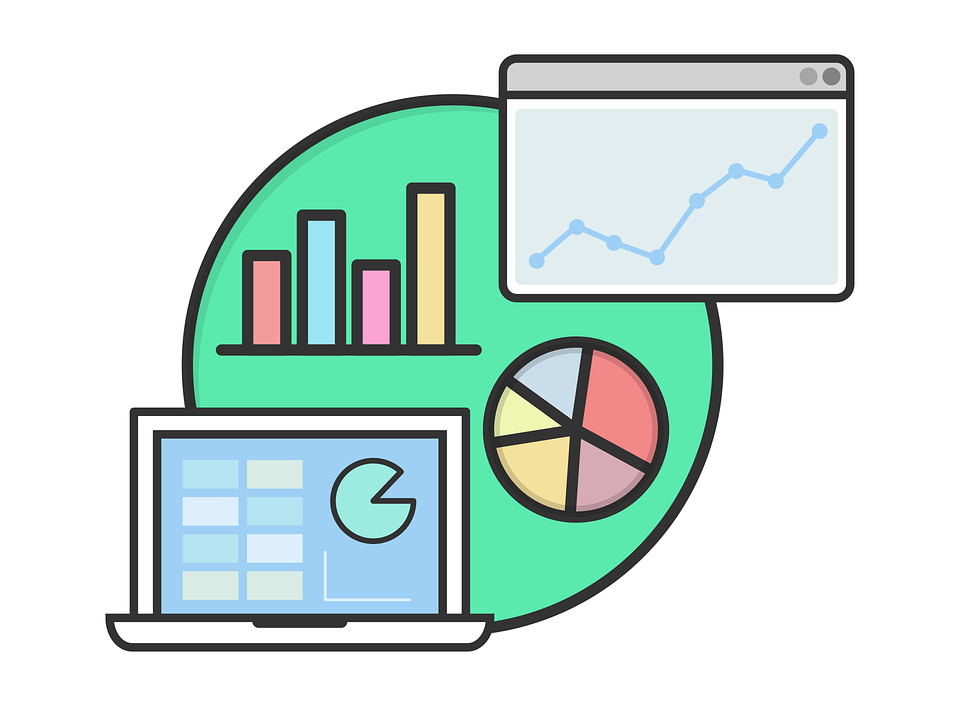5 Advanced Excel Formulas Every Professional Should Know - Blog | Excelly-AI
19 January 2023

# 5 Advanced Excel Formulas Every Professional Should Know

## Take your Excel skills to the next level with these 5 advanced formulas - VLOOKUP, INDEX, MATCH, IF and SUMIFS to solve complex problems and streamline your workflow.Excel is a powerful tool for data analysis, but to truly unlock its potential, it’s important to know some of the more advanced formulas. These formulas can help you perform complex calculations, analyze data, and make better decisions. In this article, we’ll take a look at 5 advanced Excel formulas that every professional should know.

1. IF(): The IF() formula is one of the most basic logical formulas in Excel. It allows you to perform conditional tests on data and return a specific result based on the outcome. For example, you can use the IF() formula to check if a cell value is greater than or equal to a certain number, and return “Yes” if it is, and “No” if it isn’t.

2. VLOOKUP(): The VLOOKUP() formula allows you to search for a specific value in a table and return a corresponding value from another column in that table. This can be useful for cross-referencing data from different sources, or for pulling data from a large table.

3. COUNTIF(): The COUNTIF() formula allows you to count the number of cells in a range that meet a specified criteria. For example, you can use COUNTIF() to count the number of cells in a range that contain a specific value or that are greater than a certain number.

4. SUMIF(): The SUMIF() formula is similar to COUNTIF(), but it allows you to sum the values in a range that meet a specified criteria. This can be useful for calculating totals or sub-totals based on certain conditions.

5. INDEX() & MATCH(): The INDEX() and MATCH() formulas are often used together to create a powerful tool for data analysis. The INDEX() formula returns a value from a specific cell in a range, while the MATCH() formula returns the position of a specific value within a range. Together, these formulas can be used to look up data in a table and return specific values based on a specified criteria.

In conclusion, knowing these advanced Excel formulas can help you perform more complex calculations and analyze data more effectively. They are widely used in various fields, from finance to marketing and beyond. By mastering these formulas, you’ll be able to work with Excel more efficiently and make better decisions based on your data.